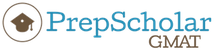## If the volume of a cube with side of length 6 is equal

##### This topic has expert replies
Legendary Member
Posts: 1763
Joined: 14 Oct 2017
Followed by:3 members

### If the volume of a cube with side of length 6 is equal

by VJesus12 » Thu Mar 15, 2018 4:13 am
If the volume of a cube with side of length 6 is equal to the volume of a rectangular solid with length 12 and width 9, then the height of the rectangular solid is

(A) 2
(B) 4
(C) 6
(D) 8
(E) 9

The OA is A.

What is the formula that I should use? And what are the equations needed?

### GMAT/MBA Expert

Legendary Member
Posts: 503
Joined: 20 Jul 2017
Thanked: 86 times
Followed by:14 members
GMAT Score:770
by ErikaPrepScholar » Thu Mar 15, 2018 5:46 am
To solve, we should know a couple volume formulas. To find volume of a cube, V = s^3, where s is the length of one side. To find volume of a rectangular solid, V = lwh, where l is length, w is width, and h is height.

We know that the volume of the cube is equal to the volume of the rectangular solid, so
s^3 = lwh

We also know that s = 6, l = 12, and w = 9, so
6^3 = (12)(9)h

We solve for h:
216 = 108h
h = 2

which gives is the height of the rectangular solid, or answer choice A.Erika John - Content Manager/Lead Instructor
https://gmat.prepscholar.com/gmat/s/

Get tutoring from me or another PrepScholar GMAT expert: https://gmat.prepscholar.com/gmat/s/tutoring/

Learn about our exclusive savings for BTG members (up to 25% off) and our 5 day free trial

Check out our PrepScholar GMAT YouTube channel, and read our expert guides on the PrepScholar GMAT blog

Legendary Member
Posts: 2184
Joined: 07 Sep 2017
Thanked: 6 times
Followed by:4 members
by Vincen » Thu Mar 15, 2018 6:51 am
Hello Vjesus12.

We know that the side of the cube is 6, and the volume of a cube is $$V_{cube}=L^3\ \ \ \ \ \Rightarrow\ \ \ \ V_{cube}=\ 6^3=216.$$ Now, this volume is equal to the volume of a rectangular solid with length 12 and width 9. The volume of this rectangular solid is $$V_{\text{rectangular}}=L\cdot W\cdot H=12\cdot9\cdot H=108H.$$ Hence we can set the equation: $$108H\ =216\ \Rightarrow\ H=2.$$ Therefore, the correct answer is the option A.

I hope it helps.

Legendary Member
Posts: 2663
Joined: 14 Jan 2015
Location: Boston, MA
Thanked: 1153 times
Followed by:127 members
GMAT Score:770
by [email protected] » Thu Mar 15, 2018 8:18 am
VJesus12 wrote:If the volume of a cube with side of length 6 is equal to the volume of a rectangular solid with length 12 and width 9, then the height of the rectangular solid is

(A) 2
(B) 4
(C) 6
(D) 8
(E) 9

The OA is A.

What is the formula that I should use? And what are the equations needed?
There's no getting around needing the equations that both Vincen and Erika used in their elegant solutions. If you were hoping to avoid doing arithmetic, once you were here: 6*6*6 = 12*9*h, you could proceed like this
6*6*6 = 2*3 * 2*3 * 2*3 = 2*2*2*3*3*3
12*9*h = 2*2*3*3*3*h
Now we have 2*2*2*3*3*3 = 2*2*3*3*3*h. All we're missing on the right side of the equation is a 2. The answer is A
Veritas Prep | GMAT Instructor

Veritas Prep Reviews
Save \$100 off any live Veritas Prep GMAT Course

### GMAT/MBA Expert

GMAT Instructor
Posts: 6195
Joined: 25 Apr 2015
Location: Los Angeles, CA
Thanked: 43 times
Followed by:24 members
by [email protected] » Mon Mar 19, 2018 5:44 am
VJesus12 wrote:If the volume of a cube with side of length 6 is equal to the volume of a rectangular solid with length 12 and width 9, then the height of the rectangular solid is

(A) 2
(B) 4
(C) 6
(D) 8
(E) 9
The volume of the cube with a side length of 6 is 6 x 6 x 6.

If we let the height of the rectangular solid = h, then we have:

6 x 6 x 6 = 12 x 9 x h

216 = 108h

2 = h

Legendary Member
Posts: 1918
Joined: 29 Oct 2017
Followed by:6 members
by swerve » Mon Mar 19, 2018 9:53 am
The volume of the cube with a side length of 6 is 6 x 6 x 6.

If we let the height of the rectangular solid = h, then we have:

6 x 6 x 6 = 12 x 9 x h

216 = 108h

2 = h. Option A.

Regards!

• Page 1 of 1